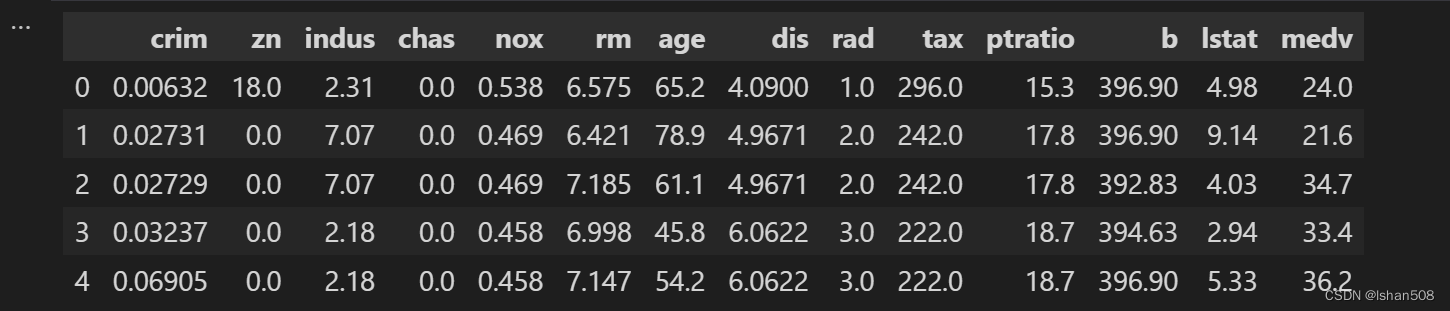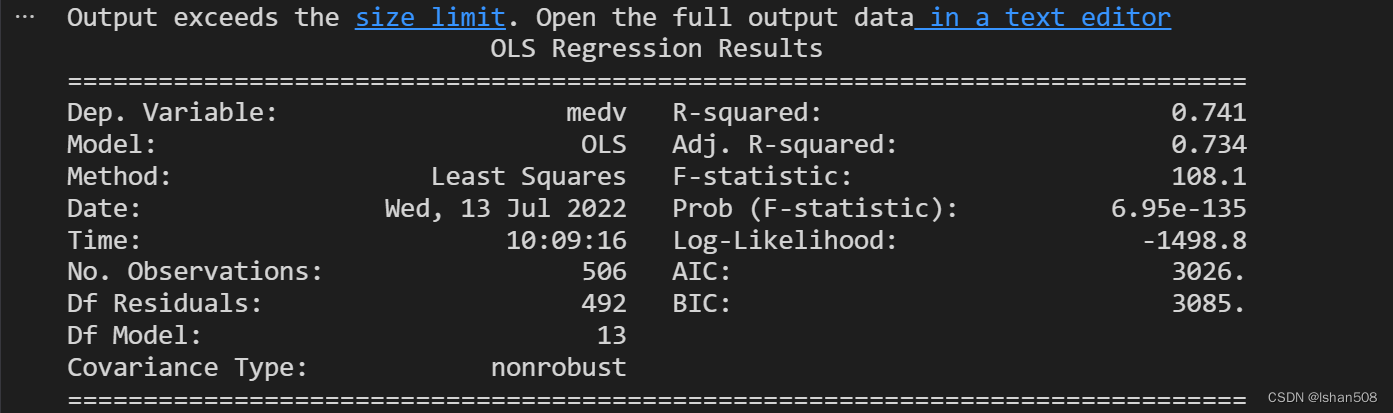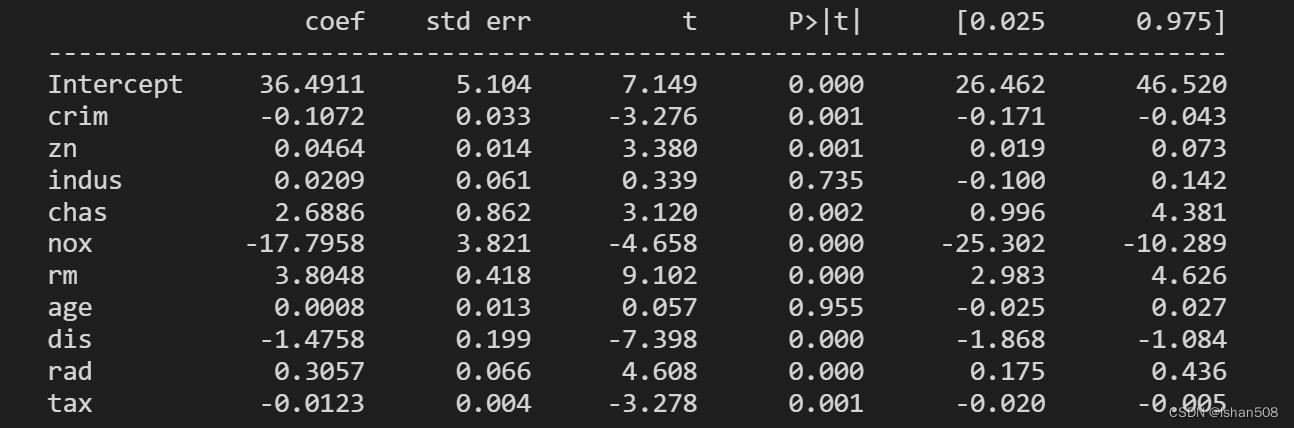# 二、多元线性回归

## 1、导入库

``import numpy as npimport pandas as pdfrom sklearn.datasets import load_boston ``

## 2.读入数据

``# 读取网络数据boston = load_boston()# 数据包含14个字段，boston.data是前13个字段数据，boston.target是第13个字段'medv'的数据col = ['crim','zn','indus','chas','nox','rm','age','dis','rad','tax','ptratio','b','lstat']bostondf = pd.DataFrame(boston.data,columns=col)bostondf['medv']=boston.targetbostondf.head()``## 3、建立回归模型

``#多元回归分析import statsmodels.formula.api as smfmod = smf.ols(formula='medv~crim+zn+indus+chas+nox+rm+age+dis+rad+tax+ptratio+b+lstat',data=bostondf)res = mod.fit()print(res.summary())``

#从回归结果可以看出回归方程的可决系数是0.741，调整可决系数是0.734，F检验的P值远小于0.05，可以认为该回归方程的线性关系是显著的。对方程的每个自变量进行t检验，发现Indus和age两个自变量P值大于0.05，即这两个变量没有通过检验，他们与因变量的相关性较弱。

## 4、剔除剔除相关性较低的两个变量再进行回归分析

``mod2 = smf.ols(formula='medv~crim+zn+chas+nox+rm+dis+rad+tax+ptratio+b+lstat',data=bostondf)res2 = mod2.fit()print(res2.summary())``